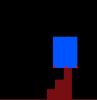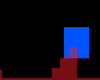# GMS 2[Solved] Tilemap-Based Slope Collision Bug

#### Fulbo

##### Member
Hi everyone!
So I'm working on a project to create a small Sonic engine, based on the guide written here: https://info.sonicretro.org/Sonic_Physics_Guide
I've run into a bug (one they said I would) within the Solid Tiles section, particular the 'Slopes & Curves' part. I'm using tiles for my terrain, height masks to calculate collision, and bbox_bottom to actually collide.

Similar in the tutorial, I check the left, right & middle corners of bbox_bottom to determine if I'd collide with a tile, and if so, snap them to the top of it. However, there are instances where none of them connect with the tile I'm currently on, so they just fall into the one below, as shown here:- Middle connects, ergo collision.- None connects.

I'm wondering if I need to instead check every pixel of bbox_bottom, and see if any of them connect to a tile. Or, is there another method I could use to handle this issue?

My code is based off on Shaun Spaulding's (top lad) tutorial on Tilemap Slope Collisions, found here:

Anyways, here's what my project contains:

Player
Code:
``````Create Event:
// Collisions
tilemap = layer_tilemap_get_id("Collisions");

// Movement
xMove = 0;
yMove = 0;
yMove_Frac = 0;

//Constants
grv = 1;
horSpeed = 4;
jmp = 6;``````
Code:
`````` Step Event:
// Current Positions Before Movement
var oldBBotLeft = (scr_inFloor(tilemap, bbox_left, bbox_bottom + 1) >= 0);
var oldBBot = (scr_inFloor(tilemap, x, bbox_bottom + 1) >= 0);
var oldBBotRight = (scr_inFloor(tilemap, bbox_right, bbox_bottom + 1) >= 0);

// Movement
xMove = (keyboard_check(vk_right) - keyboard_check(vk_left)) * horSpeed;

// Collision Hor
var bbox_side;
if (xMove > 0) bbox_side = bbox_right; else bbox_side = bbox_left;

var p1 = tilemap_get_at_pixel(tilemap, bbox_side + xMove, bbox_top);
var p2 = tilemap_get_at_pixel(tilemap, bbox_side + xMove, bbox_bottom);

// If middle centre is in a solid tile...
if (tilemap_get_at_pixel(tilemap, x, bbox_bottom) > 1) p2 = 0; // Ignore bottom side tiles if on a slope

// Actually Collide
if (p1 == 1 || p2 == 1) {    // Don't set check to 'p != 0', otherwise you won't be able to climb up slopes

if (xMove > 0) { x = x - (x mod TILE_SIZE) + (TILE_SIZE - 1) - (bbox_right - x) }
else { x = x - (x mod TILE_SIZE) - (bbox_left - x); }

xMove = 0;
}

// Move X
x += xMove;

// BBox_Bottom Position Recordings
var curBBotLeft = (scr_inFloor(tilemap, bbox_left, bbox_bottom + 1) >= 0);
var curBBot = (scr_inFloor(tilemap, x, bbox_bottom + 1) >= 0);
var curBBotRight = (scr_inFloor(tilemap, bbox_right, bbox_bottom + 1) >= 0);

// Jump
if (curBBotLeft || curBBot || curBBotRight) {

// Jump
if (keyboard_check_pressed(ord("Z"))) { yMove = -jmp; }

} else { // If in air...

// Gravity
if (yMove < 6) { yMove += grv;} // Increase Gravity
else if (yMove > 6) { yMove = 6; } // Terminal Velocity
}

// Store Fractions
var nYMove = yMove;
nYMove += yMove_Frac;

// Convert Decimal to Integer With Fractions
yMove_Frac = nYMove - (floor(abs(nYMove)) * sign(nYMove));
nYMove -= yMove_Frac;

// Collision Vert
var nextBBotLeft = tilemap_get_at_pixel(tilemap, bbox_left, bbox_bottom + nYMove);
var nextBBot = tilemap_get_at_pixel(tilemap, x, bbox_bottom + nYMove);
var nextBBotRight = tilemap_get_at_pixel(tilemap, bbox_right, bbox_bottom + nYMove);

// Prepare for Slope Collision
var floorOffset; floorOffset = scr_inFloor(tilemap, bbox_left, bbox_bottom + nYMove);    // Bottom Left
floorOffset = scr_inFloor(tilemap, x, bbox_bottom + nYMove);            // Bottom
floorOffset = scr_inFloor(tilemap, bbox_right, bbox_bottom + nYMove);    // Bottom Right

// Determine What BBox_Bottom Corner is Closest to a Floor
var leftCloser =    (floorOffset >= floorOffset && floorOffset >= floorOffset);
var middleCloser =  (floorOffset >= floorOffset && floorOffset >= floorOffset);
var rightCloser =    (floorOffset >= floorOffset && floorOffset >= floorOffset);

if ((nextBBot != 0 || nextBBotLeft != 0 || nextBBotRight != 0)) { // If you'll collide into a tile...

/// Move Up Along Slopes
if (floorOffset >= 0 || floorOffset >= 0 || floorOffset >= 0) { // If you'll collide into a height mask/pixel...

// Snap to the top of the height mask

// Bottom Left
if (floorOffset >= 0 && leftCloser) { // If bottom left colliding, && offset higher than bottom right...
y -= (floorOffset + 1);

// If you're inside a tile...
while (scr_inFloor(tilemap, bbox_left, bbox_bottom + yMove) >= 0) { // + yMove assures this works.
y--; } }

// Bottom
else if (floorOffset >= 0 && middleCloser) { // If bottom right colliding, && offset higher than bottom left..
y -= (floorOffset + 1);    // floorOffset = -1; }

// If you're inside a tile...
while (scr_inFloor(tilemap, x, bbox_bottom + yMove) >= 0) { // + yMove assures this works.
y--; } }

// Bottom Right
else if (floorOffset >= 0 && rightCloser) { // If bottom right colliding, && offset higher than bottom left..
y -= (floorOffset + 1);    // floorOffset = -1; }

// If you're inside a tile...
while (scr_inFloor(tilemap, bbox_right, bbox_bottom + yMove) >= 0) { // + yMove assures this works.
y--; } }

yMove = 0;
inAir = false;
}
}

// Move Y
y += nYMove;

// Move Down Along Slopes
var yLen = 9; // The max distance from a tile you can be before you snap to it.

// Check if you were on a tile in the last frame, and are currently airborne.
if ((oldBBotLeft || oldBBotRight) && yMove > 0) {

var bBotLeftLen = (scr_inFloor(tilemap, bbox_left, bbox_bottom + yLen) >= 0);
var bBotLen = (scr_inFloor(tilemap, x, bbox_bottom + yLen) >= 0);
var bBotRightLen = (scr_inFloor(tilemap, bbox_right, bbox_bottom + yLen) >= 0);

if (bBotLeftLen || bBotLen || bBotRightLen) {

if (bBotLeftLen && leftCloser)            { y += abs(floorOffset) - 1; }
else if (bBotRightLen && rightCloser)    { y += abs(floorOffset) - 1; }
}
}``````
I apologize if this is a bit hard/much to read for others. If it is, I'll fix it up later.

Anyways, if you have any suggestions, I'd be very grateful to hear them out.

#### Fulbo

##### Member
I currently can't edit my post as it contains links, so I'll post the rest of the code I didn't put in above.

scr_inFloor
Code:
``````/// @descrption Checks height-depth of a non-empty tile
/// @arg tilemap
/// @arg x
/// @arg y

// Video Reference (Around 20:10): https://www.youtube.com/watch?v=Yre-XAE8DBA

// Below system won't work if mirror_flipping tiles.

var pos = tilemap_get_at_pixel(argument0, argument1, argument2);

// tile_get_index(pos); // Useful for mirror_flipped tiles. Best look this one up.

if (pos > 0) { // If the tile is solid...

if (pos == 1) { // If literally inside a tile...
return (argument2 mod TILE_SIZE); } // Returns how deep into a tile you are (before hitting a coloured pixel)

var theFloor = global.heights[(argument1 mod TILE_SIZE) + (pos * TILE_SIZE)];

// Return the difference between our tile depth and the actual height of the tile
return ((argument2 mod TILE_SIZE) - theFloor);

// If above tile (pos <= -1), return how far we are above it
} else { return -(TILE_SIZE - (argument2 mod TILE_SIZE)); }``````
Also, as per Shaun's Tutorial, I created an object responsible for setting up the tiles, and getting their height mask data. Here's what I have:

obj_tileSetup
Code:
`````` Create Event:

/// @description Setup Tile Data

// Video Explanation (round 8:07): https://www.youtube.com/watch?v=Yre-XAE8DBA

#macro TILE_SIZE 16 // Don't end macros with ';'

// How many columns we need to check for height data
heightsToGetW = sprite_get_width(sheet_cols);

// How many rows we need to check for height data
heightsToGetH = sprite_get_height(sheet_cols);

// Return the number of collision types in the tileSheet
tilesCol = heightsToGetW / TILE_SIZE;
tilesRow = heightsToGetH / TILE_SIZE;

// Setup New TileLayer
layerid = layer_create(0, "DynamicCollisions");
tilemapid = layer_tilemap_create(layerid, 0, 0, tiles_cols, tilesCol, tilesRow); // width: How many tiles. height: 1 height = 1 tile high. 2 height = 2 tiles high.

// Create Tiles to be Used
for (var i = 0; i <= tilesCol; i++) {

for (var j = 0; j <= tilesRow; j++) {

tilemap_set(tilemapid, i, i, j); // Change cell_y to a dynamic variable, if tile_cols has more than 1 row.

}
}``````
Code:
`````` Draw Event:

/// @description Build Height Table Then Start Game

draw_tilemap(tilemapid, 0, 0); // Draw tilemap early.

// Record Height of Each Column
for (var i = heightsToGetW - 1; i >= 0; i--) { // Furthest tile to nearest tile.

var blankRows = 0;
var emptyColour = c_black;
while (blankRows <= TILE_SIZE) {

// Record in Global Array
global.heights[i] = blankRows;
if (blankRows == TILE_SIZE) { break; } // Stops program checking underneath a tile.

// Stop Checking Once Pixel-Data is Found
if (surface_getpixel(application_surface, i, blankRows) != emptyColour) { break; } // Basically returns the color in the current cell (including blank).

blankRows++; // If cell blank, increment.
}
}

// Hide Drawn Tiles From Users
room_goto_next();``````

#### TheouAegis

##### Member
You just need to add another point. The distance between points can be no larger than your tile width. Your sprite is clearly more than double the tile size, so you need more points to check.

#### Fulbo

##### Member
You just need to add another point. The distance between points can be no larger than your tile width. Your sprite is clearly more than double the tile size, so you need more points to check.
Yeah, that's a better idea than check all spaces. I'm thinking of just placing two checks between left - middle & middle - right, so there's only a 4 pixel difference instead of 8. Hopefully that'll work fine. (If it does, I'll change help to solved.)

Edit: So the collision works nicely know, and I'm not clipping through the tile anymore. I'm thinking thought it's only working now because how the level's setup, where the player is and how they move. A different level layout might cause issues.
I'll keep the code as is for now, by I may implement something more robust in the future. Apologies for asking again, but do you know of a better code setup I look into?

Last edited:

#### TheouAegis

##### Member
Not really. Shaun's sloped tiles tutorial was one of his tutorials I actually liked. It does have some minor issues and should have covered optimizations, but it's a good method.

#### Fulbo

##### Member
Okay then. Spose it'll be good to work with some limitations, and try to optimize things when I can.
Thanks for your responses, Theo. Cheers.